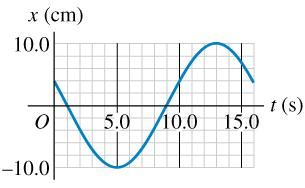# Problem: The displacement of an oscillating object as a function of time is shown in the figure.a)What is the frequency?b)What is the amplitude?c)What is the period?d)What is the angular frequency of this motion?

###### FREE Expert Solution

(a)

Frequency, f = 1/T

Periodic time, T = 16.0s

90% (210 ratings)###### Problem DetailsThe displacement of an oscillating object as a function of time is shown in the figure.

a)What is the frequency?

b)What is the amplitude?

c)What is the period?

d)What is the angular frequency of this motion?